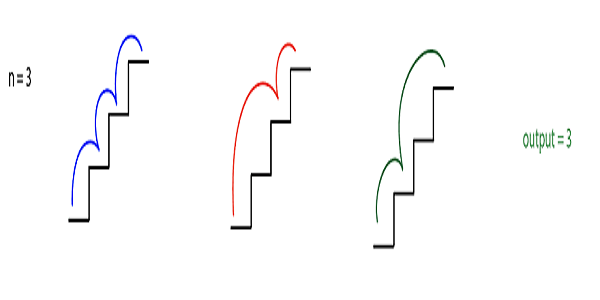9

# Count Ways To Reach The N-th Stairs

Difficulty: MEDIUM
Avg. time to solve
30 min
Success Rate
80%

Problem Statement
Suggest Edit

#### You have been given a number of stairs. Initially, you are at the 0th stair, and you need to reach the Nth stair. Each time you can either climb one step or two steps. You are supposed to return the number of distinct ways in which you can climb from the 0th step to Nth step.

##### Example :
``````N=3
````````````We can climb one step at a time i.e. {(0, 1) ,(1, 2),(2,3)} or we can climb the first two-step and then one step i.e. {(0,2),(1, 3)} or we can climb first one step and then two step i.e. {(0,1), (1,3)}.
``````

#### Input format :

``````The first line contains an integer 'T', which denotes the number of test cases or queries to be run. Then the test cases follow.

The first and the only argument of each test case contains an integer 'N', representing the number of stairs.
``````
##### Output format :
``````For each test case/query, print the number of distinct ways to reach the top of stairs. Since the number can be huge, so return output modulo 10^9+7.

Output for every test case will be printed in a separate line.
``````
##### Note :
``````You do not need to print anything. It has already been taken care of.
``````
##### Constraints :
``````1 <= T <= 100
0 <= N <= 10^18

Where 'T' is the number of test cases, and 'N' is the number of stairs.
``````
##### Sample Input 1 :
``````2
2
3
``````
##### Sample Output 1 :
``````2
3
``````
##### Explanation Of Sample Input 1 :
``````In the first test case, there are only two ways to climb the stairs, i.e. {1,1} and {2}.

In the second test case, there are three ways to climb the stairs i.e. {1,1,1} , {1,2} and {2,1}.
``````
##### Sample Input 2 :
``````2
4
5
``````
##### Sample Output 2 :
``````2
3
``````
##### Explanation Of Sample Input 2 :
``````In the first test case, there are five ways to climb the stairs i.e. {1,1,1,1} , {1,1,2} , {2,1,1} , {1,2,1} , {2,2}.

In the second test case, there are eight ways to climb the stairs i.e. {1,1,1,1,1} , {1,1,1,2} , {1,1,2,1}, {1,2,1,1}, {2,1,1},{2,2,1},{2,1,2} and {2,2,1}.
``````Console